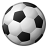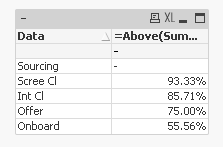# QlikView App Dev

Discussion Board for collaboration related to QlikView App Development.

Announcements
Coming Aug. 9: New Simplified Authoring for Qlik Sense SaaS – For Details, CLICK HERE
cancel
Showing results for
Did you mean:Contributor

## Sum of values below divided by sum of values one cell above and below

Hi,

I have an excel file which has data like below

 Data Cleared Status % Calc Sourcing 10 0% Scree Cl 20 0.933333 Int Cl 30 0.857143 Offer 40 0.75 Onboard 50

% calc is field I need to derive in qlikview.

The way it is calculated as is

(20+30+40+50)/(10+20+30+40+50)=0.933.. similarly (30+40+50)/(20+30+40+50)=0.8571.

I tried different combination of Rangesum function but it didn't work.

1 Solution

Accepted SolutionsMVP

May be this

=Above(Sum(TOTAL [Cleared Status]) - RangeSum(Above(Sum([Cleared Status]), 0, RowNo())))/Above(Sum(TOTAL [Cleared Status]) - RangeSum(Above(Sum([Cleared Status]), 1, RowNo())))2 RepliesMVP

May be this

=Above(Sum(TOTAL [Cleared Status]) - RangeSum(Above(Sum([Cleared Status]), 0, RowNo())))/Above(Sum(TOTAL [Cleared Status]) - RangeSum(Above(Sum([Cleared Status]), 1, RowNo())))Contributor
Author

Perfect..Thanks